# 関数プログラミングことはじめ in 福岡

Jan. 20, 2019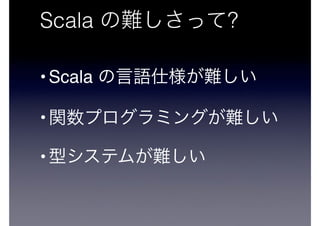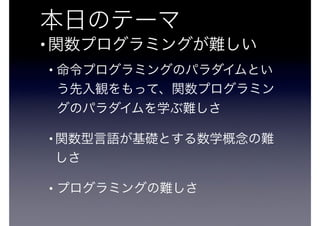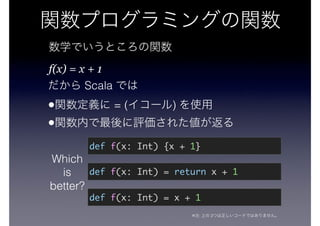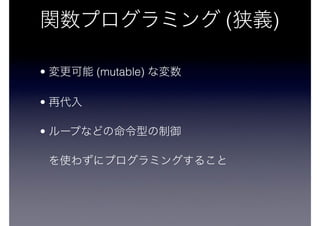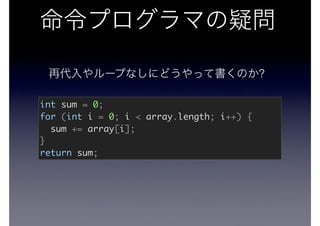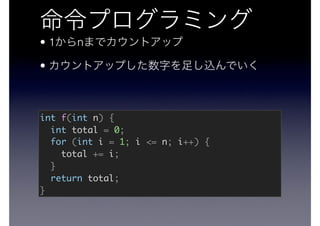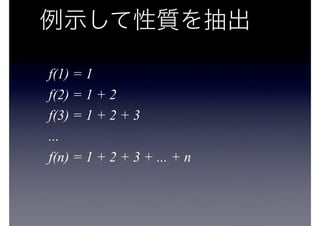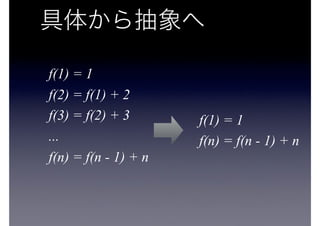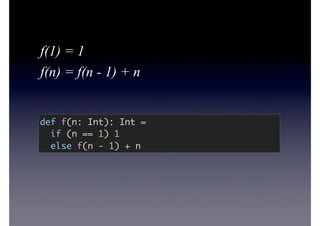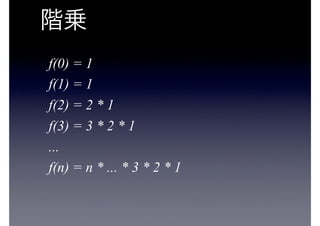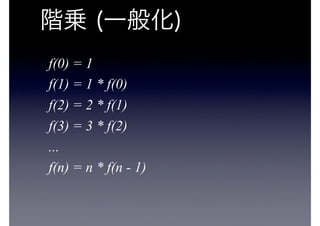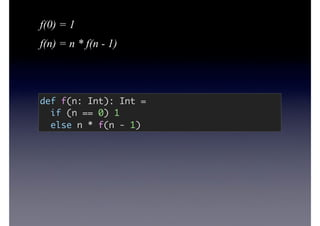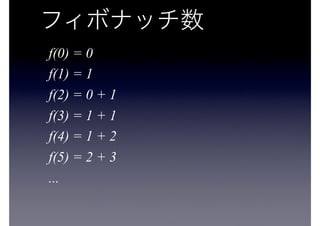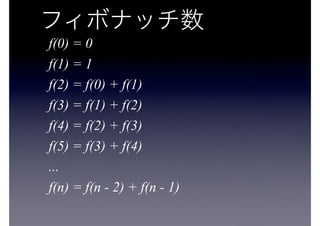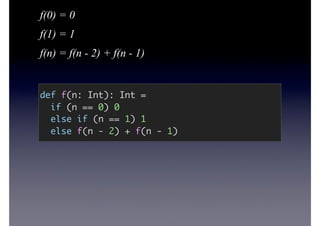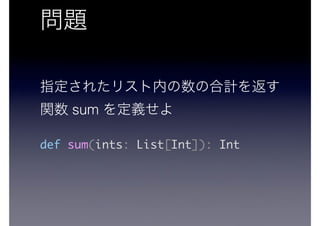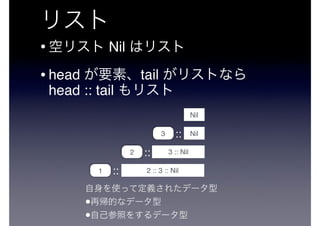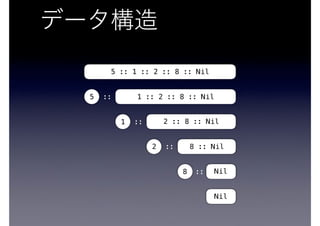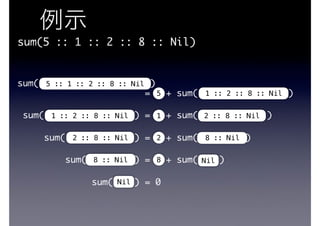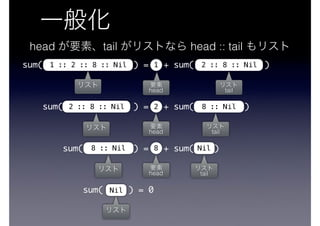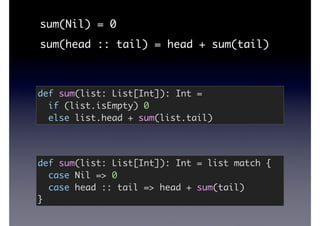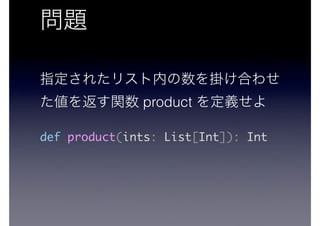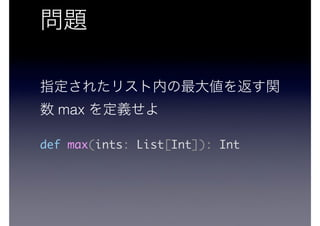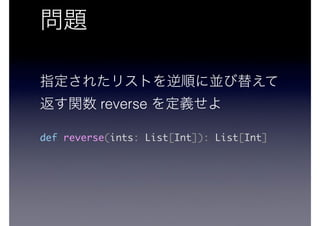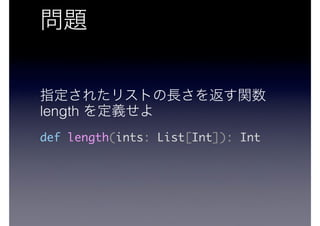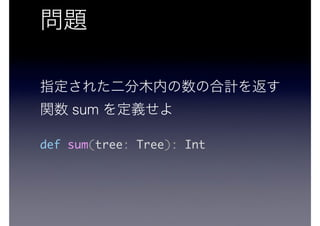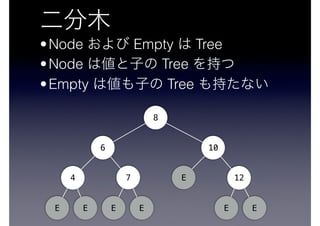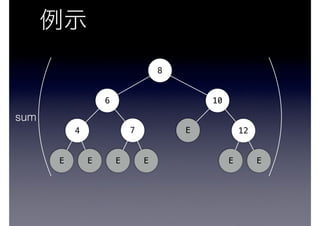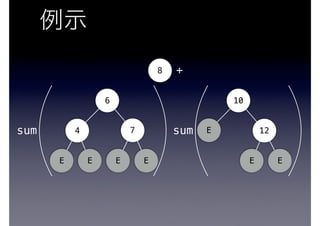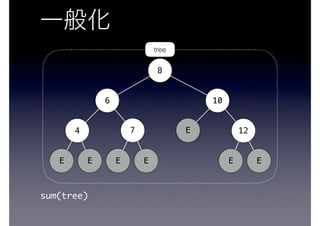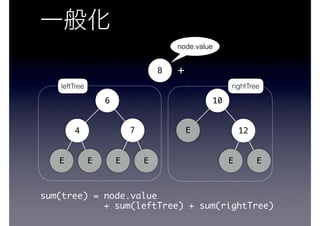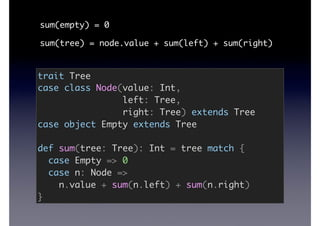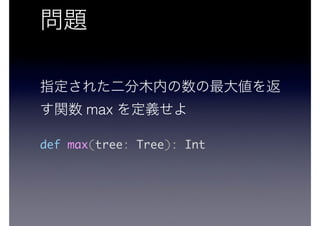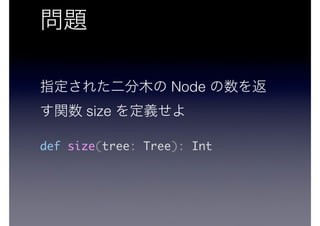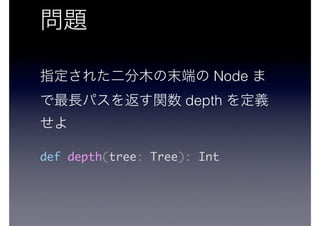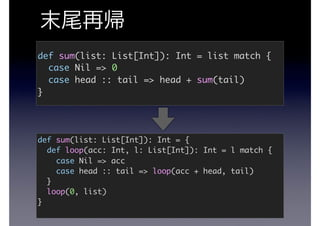1 of 55

### 関数プログラミングことはじめ in 福岡

1. in Scala Fukuoka 2019 https://goo.gl/iKyEKs
2. Scala
3. Scala ?
4. ?
5. •Scala • • Scala ?
6. • • • •
7. • • • •
8. f(x) = x + 1 Scala • = ( ) • Which is better? def f(x: Int) {x + 1} def f(x: Int) = x + 1 def f(x: Int) = return x + 1 ※ : 2
9. ( ) • (mutable) • •
10. ? int sum = 0; for (int i = 0; i < array.length; i++) { sum += array[i]; } return sum;
11. https://goo.gl/iKyEKs
12. • 1 n • int f(int n) { int total = 0; for (int i = 1; i <= n; i++) { total += i; } return total; }
13. f(1) = 1 f(2) = 1 + 2 f(3) = 1 + 2 + 3 ... f(n) = 1 + 2 + 3 + ... + n
14. f(1) = 1 f(2) = f(1) + 2 f(3) = f(2) + 3 ... f(n) = f(n - 1) + n f(1) = 1 f(n) = f(n - 1) + n
15. def f(n: Int): Int = if (n == 1) 1 else f(n - 1) + n f(1) = 1 f(n) = f(n - 1) + n
16. f(0) = 1 f(1) = 1 f(2) = 2 * 1 f(3) = 3 * 2 * 1 ... f(n) = n * ... * 3 * 2 * 1
17. f(0) = 1 f(1) = 1 * f(0) f(2) = 2 * f(1) f(3) = 3 * f(2) ... f(n) = n * f(n - 1)
18. f(0) = 1 f(n) = n * f(n - 1) def f(n: Int): Int = if (n == 0) 1 else n * f(n - 1)
19. n ? 1, 1, 2, 3, 5, 8, 13, …
20. f(0) = 0 f(1) = 1 f(2) = 0 + 1 f(3) = 1 + 1 f(4) = 1 + 2 f(5) = 2 + 3 ...
21. f(0) = 0 f(1) = 1 f(2) = f(0) + f(1) f(3) = f(1) + f(2) f(4) = f(2) + f(3) f(5) = f(3) + f(4) ... f(n) = f(n - 2) + f(n - 1)
22. f(0) = 0 f(1) = 1 f(n) = f(n - 2) + f(n - 1) def f(n: Int): Int = if (n == 0) 0 else if (n == 1) 1 else f(n - 2) + f(n - 1)
23. sum def sum(ints: List[Int]): Int
24. • Nil •head tail head :: tail 3 Nil Nil:: 3 :: Nil2 :: 2 :: 3 :: Nil1 :: • •
25. Nil Nil::8 8 :: Nil::2 2 :: 8 :: Nil::1 1 :: 2 :: 8 :: Nil::5 5 :: 1 :: 2 :: 8 :: Nil
26. sum(5 :: 1 :: 2 :: 8 :: Nil) sum( )  = + sum( ) sum( ) = + sum( ) sum( ) = + sum( ) sum( ) = + sum( ) sum( ) = 0Nil 8 :: Nil 2 :: 8 :: Nil 5 :: 1 :: 2 :: 8 :: Nil Nil8 8 :: Nil2 2 :: 8 :: Nil1 5 1 :: 2 :: 8 :: Nil 1 :: 2 :: 8 :: Nil
27. sum( ) = + sum( ) sum( ) = + sum( ) sum( ) = + sum( ) sum( ) = 0Nil 8 :: Nil 2 :: 8 :: Nil Nil8 8 :: Nil22 :: 8 :: Nil 1 head head tail head tail head tail head :: tail 1 :: 2 :: 8 :: Nil tail
28. sum(Nil) = 0 sum(head :: tail) = head + sum(tail) def sum(list: List[Int]): Int = if (list.isEmpty) 0 else list.head + sum(list.tail) def sum(list: List[Int]): Int = list match { case Nil => 0 case head :: tail => head + sum(tail) }
29. product def product(ints: List[Int]): Int
30. max def max(ints: List[Int]): Int
31. reverse def reverse(ints: List[Int]): List[Int]
32. length def length(ints: List[Int]): Int
33. sum def sum(tree: Tree): Int
34. •Node Empty Tree •Node Tree •Empty Tree 8 6 10 4 7 E 12 E E E E E E
35. Tree Node Tree Tree 8 6 10 4 7 E 12 E E E E E E
36. 8 6 10 4 7 E 12 E E E E E E sum
37. 8 6 10 4 7 E 12 E E E E E E sum sum +
38. sum(tree) tree 8 6 10 4 7 E 12 E E E E E E
39. sum(tree) = node.value  + sum(leftTree) + sum(rightTree) leftTree rightTree + node.value 8 6 10 4 7 E 12 E E E E E E
40. sum(empty) = 0 sum(tree) = node.value + sum(left) + sum(right) trait Tree case class Node(value: Int, left: Tree, right: Tree) extends Tree case object Empty extends Tree def sum(tree: Tree): Int = tree match { case Empty => 0 case n: Node => n.value + sum(n.left) + sum(n.right) }
41. max def max(tree: Tree): Int
42. Node size def size(tree: Tree): Int
43. Node depth def depth(tree: Tree): Int
44. def sum(list: List[Int]): Int = list match { case Nil => 0 case head :: tail => head + sum(tail) } def sum(list: List[Int]): Int = { def loop(acc: Int, l: List[Int]): Int = l match { case Nil => acc case head :: tail => loop(acc + head, tail) } loop(0, list) }
45. • Functional Programming Principles in Scala  Scala Martin Odersky   https://www.coursera.org/course/progfun •   OCaml   http://www.amazon.co.jp/dp/4781911609
46. • Scala & ― Scalaz   2   http://www.amazon.co.jp/dp/4844337769